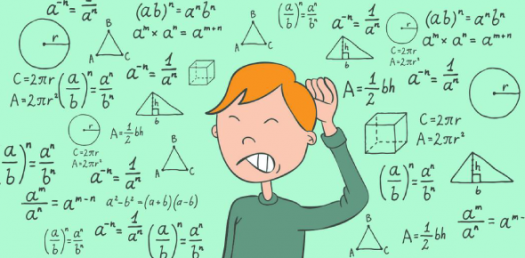# Math Test: Logarithm Trivia Quiz

15 Questions | Total Attempts: 523SettingsBelow is a math Test Logarithm Trivia Quiz. Most people don’t know how to read the logarithm tables or use a calculator to solve them. By taking this quiz, you will be a step closer when it comes to an understanding and solving logarithm problems. Do give it a try and get the practice you desire. All the best, and keep practicing.

• 1.
• A.

Real = 3 , Imaginary = -4

• B.

Real = 3 , Imaginary = 4

• C.

Real = -3 , Imaginary = 4

• D.

Real = -3 , Imaginary = -4

• 2.
• A.

X = 0.75

• B.

X = 0.74

• C.

X = 0.73

• D.

X = 0.72

• 3.
• A.
• B.
• C.
• D.
• 4.
• A.

(-2, 3)

• B.

(-2, -3)

• C.

(2, 3)

• D.

(2, -3)

• 5.
• A.

(-0.5, 0.5)

• B.

(-0.5,- 0.5)

• C.

(0.5, -0.5)

• D.

(0.5, 0.5)

• 6.
• A.

1.40

• B.

1.41

• C.

1.42

• D.

1.43

• 7.
• A.

A =1, b = 1, c = 2

• B.

A =1, b = -1, c = -2

• C.

A =1, b = 1, c = -2

• D.

A =-1, b = 1, c = -2

• 8.
From Question 7, Factorize P(x) completely.
• A.

(x-3) (x-1) (x+2)

• B.

(x-3) (x-1) (x-2)

• C.

(x+3) (x-1) (x+2)

• D.

(x-3) (x+1) (x+2)

• 9.
From Question 7, State all the zeros of P(x)
• A.

Zeroes: 3,-1, -2

• B.

Zeroes: 3,1, 2

• C.

Zeroes: 3,1, -2

• D.

Zeroes: -3,1, -2

• 10.
• A.
• B.
• C.
• D.
• 11.
• A.

Gf(x) = fg(x) = 1- x

• B.

Gf(x) = fg(x) = 2x

• C.

Gf(x) = fg(x) = -x

• D.

Gf(x) = fg(x) = x

• 12.
From Question 11, state the domain and range for f and g
• A.

Df = Rg = [3, ∞); Rf = Dg = [5, +∞)]

• B.

Df = Rg = [3, -∞); Rf = Dg = [5, +∞)]

• C.

Df = Rg = [-3, ∞); Rf = Dg = [5, +∞)]

• D.

Df = Rg = [3, ∞); Rf = Dg = [-5, +∞)]

• 13.
From Question 11, sketch f and g on the same axes.
• A.

Df =Rg = (-1, ∞); Rf = Dg = (1, +∞)]

• B.

Df =Rg = (-1, ∞); Rf = Dg = (-∞, 1)]

• C.

Df =Rg = (-1, ∞); Rf = Dg = (-∞, +∞)]

• D.

Df =Rg = (1, ∞); Rf = Dg = (-∞, +∞)]

• 14.
• A.
• B.
• C.
• D.
• 15.
• A.
• B.
• C.
• D.
Related TopicsBack to top